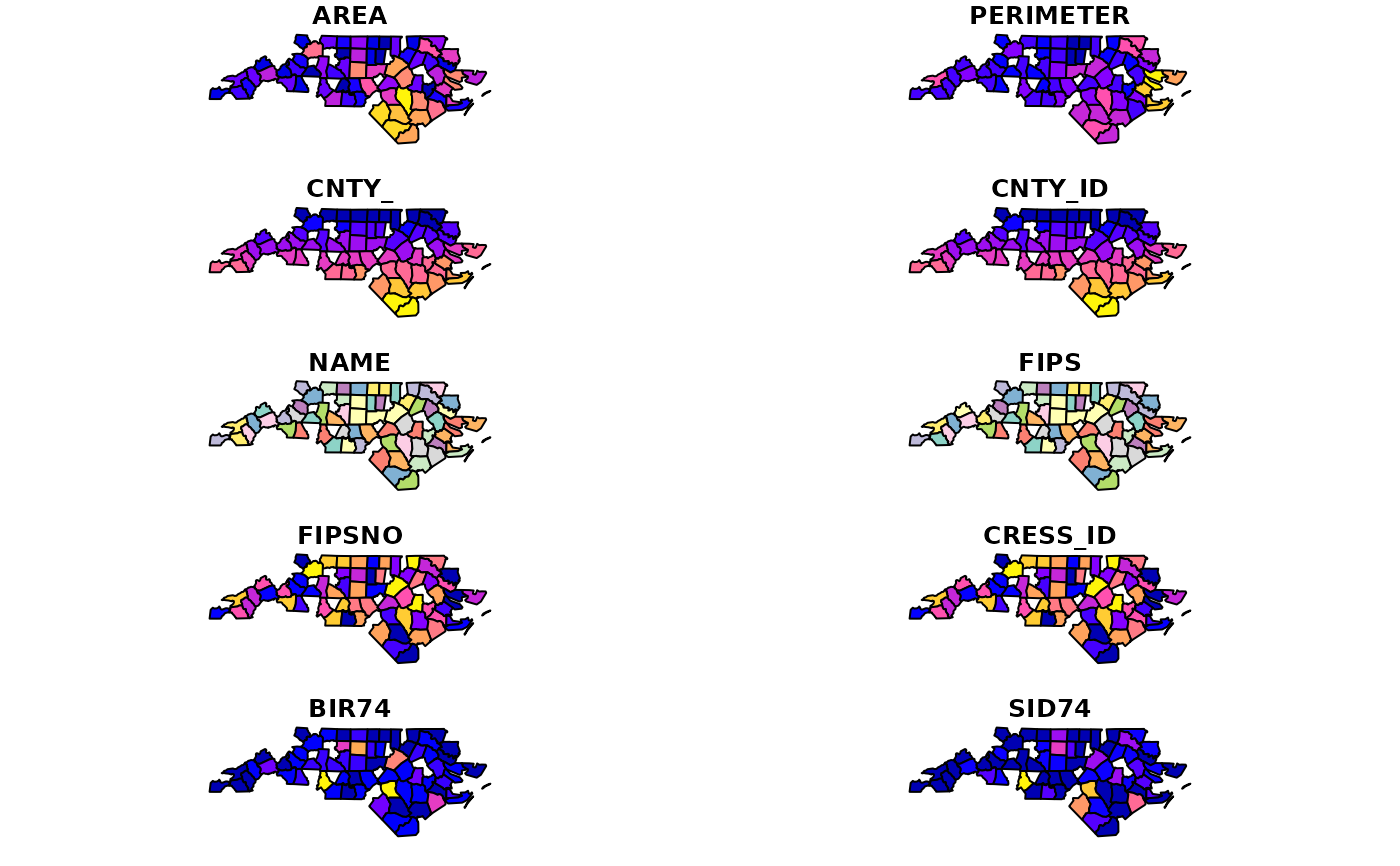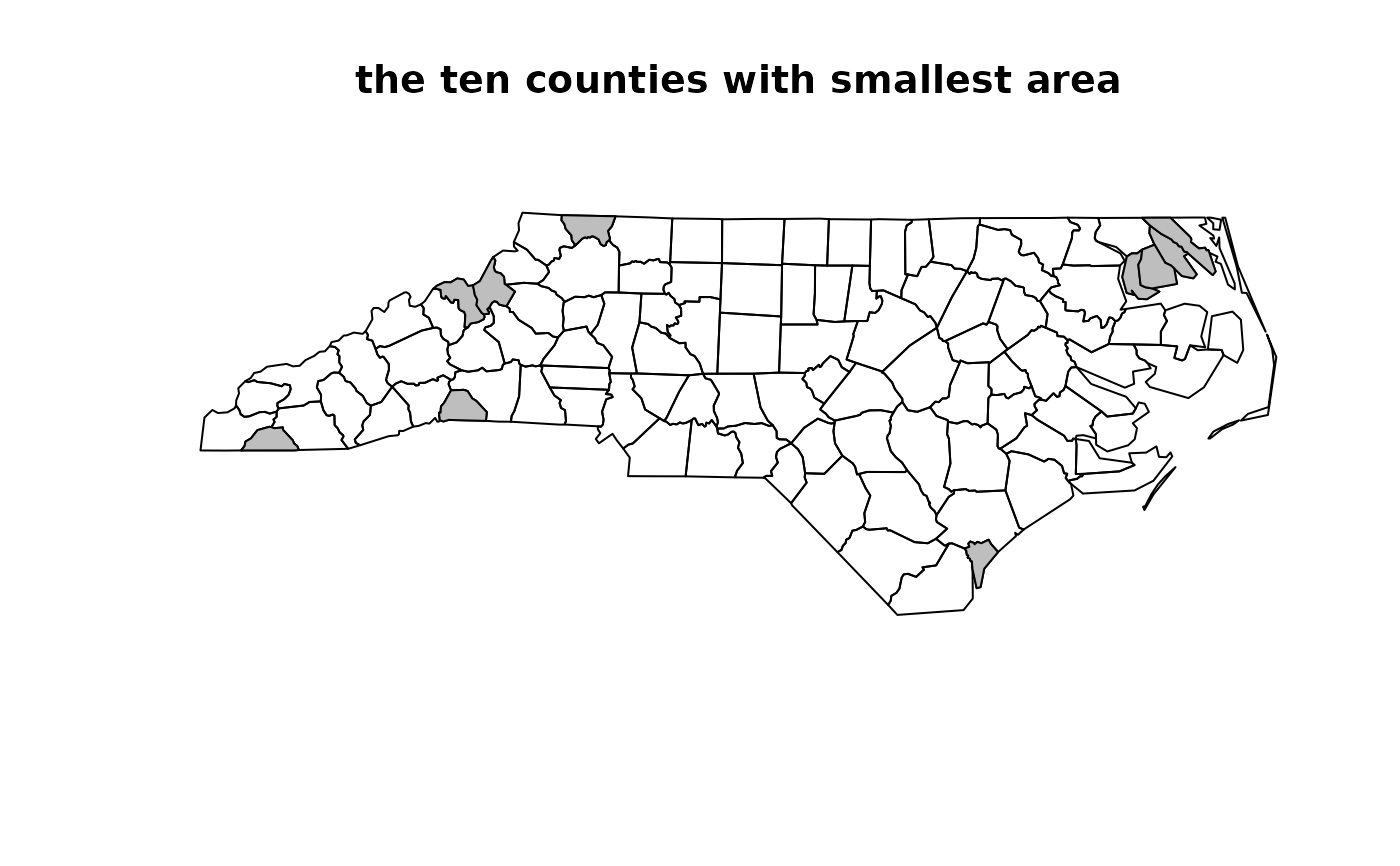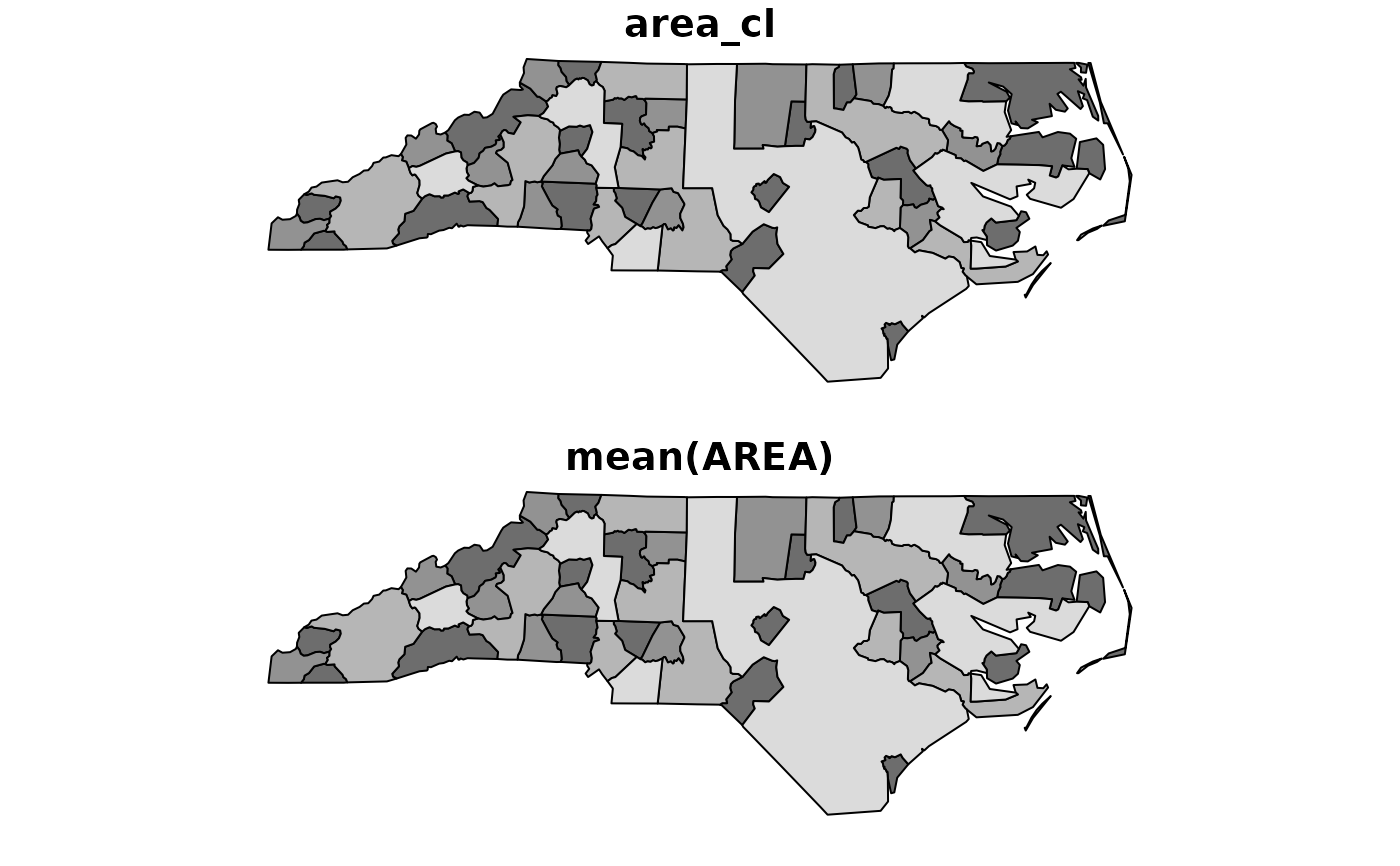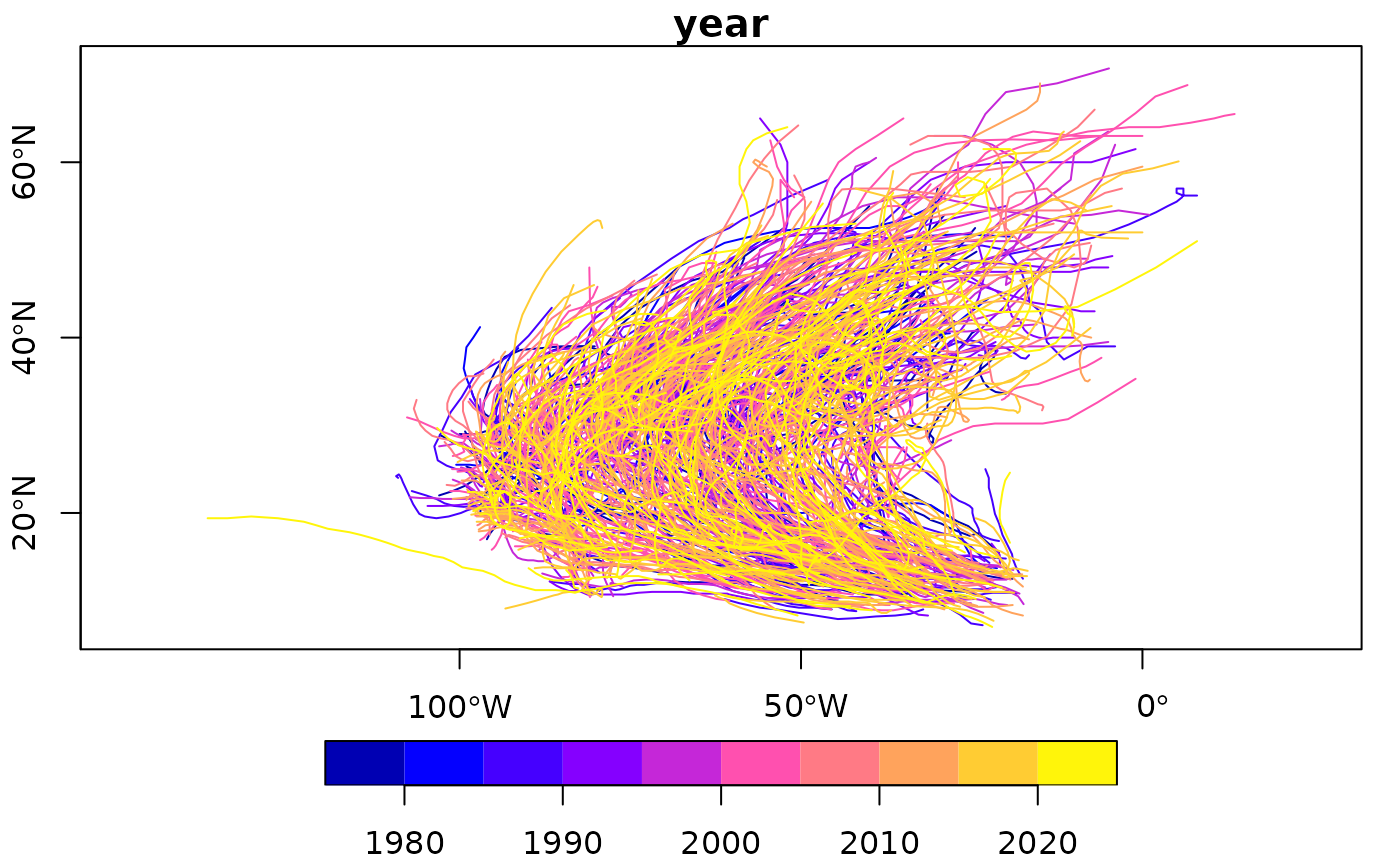Tidyverse methods for sf objects. Geometries are sticky, use as.data.frame to let dplyr's own methods drop them. Use these methods without the .sf suffix and after loading the tidyverse package with the generic (or after loading package tidyverse).

## Usage

dplyr_reconstruct.sf(data, template)

filter.sf(.data, ..., .dots)

arrange.sf(.data, ..., .dots)

ungroup.sf(x, ...)

rowwise.sf(x, ...)

mutate.sf(.data, ..., .dots)

transmute.sf(.data, ..., .dots)

select.sf(.data, ...)

rename.sf(.data, ...)

slice.sf(.data, ..., .dots)

summarise.sf(.data, ..., .dots, do_union = TRUE, is_coverage = FALSE)

distinct.sf(.data, ..., .keep_all = FALSE)

gather.sf(
data,
key,
value,
...,
na.rm = FALSE,
convert = FALSE,
factor_key = FALSE
)

pivot_longer.sf(
data,
cols,
names_to = "name",
names_prefix = NULL,
names_sep = NULL,
names_pattern = NULL,
names_ptypes = NULL,
names_transform = NULL,
names_repair = "check_unique",
values_to = "value",
values_drop_na = FALSE,
values_ptypes = NULL,
values_transform = NULL,
...
)

pivot_wider.sf(
data,
...,
id_cols = NULL,
id_expand = FALSE,
names_from = name,
names_prefix = "",
names_sep = "_",
names_glue = NULL,
names_sort = FALSE,
names_vary = "fastest",
names_expand = FALSE,
names_repair = "check_unique",
values_from = value,
values_fill = NULL,
values_fn = NULL,
unused_fn = NULL
)

data,
key,
value,
fill = NA,
convert = FALSE,
drop = TRUE,
sep = NULL
)

sample_n.sf(tbl, size, replace = FALSE, weight = NULL, .env = parent.frame())

sample_frac.sf(
tbl,
size = 1,
replace = FALSE,
weight = NULL,
.env = parent.frame()
)

nest.sf(.data, ...)

separate.sf(
data,
col,
into,
sep = "[^[:alnum:]]+",
remove = TRUE,
convert = FALSE,
extra = "warn",
fill = "warn",
...
)

separate_rows.sf(data, ..., sep = "[^[:alnum:]]+", convert = FALSE)

unite.sf(data, col, ..., sep = "_", remove = TRUE)

unnest.sf(data, ..., .preserve = NULL)

drop_na.sf(x, ...)

inner_join.sf(x, y, by = NULL, copy = FALSE, suffix = c(".x", ".y"), ...)

left_join.sf(x, y, by = NULL, copy = FALSE, suffix = c(".x", ".y"), ...)

right_join.sf(x, y, by = NULL, copy = FALSE, suffix = c(".x", ".y"), ...)

full_join.sf(x, y, by = NULL, copy = FALSE, suffix = c(".x", ".y"), ...)

semi_join.sf(x, y, by = NULL, copy = FALSE, suffix = c(".x", ".y"), ...)

anti_join.sf(x, y, by = NULL, copy = FALSE, suffix = c(".x", ".y"), ...)

## Arguments

data

see original function docs

template

see original function docs

.data

data object of class sf

...

other arguments

.dots

see corresponding function in package dplyr

see corresponding function in dplyr

x, y

A pair of data frames, data frame extensions (e.g. a tibble), or lazy data frames (e.g. from dbplyr or dtplyr). See Methods, below, for more details.

do_union

logical; in case summary does not create a geometry column, should geometries be created by unioning using st_union, or simply by combining using st_combine? Using st_union resolves internal boundaries, but in case of unioning points, this will likely change the order of the points; see Details.

is_coverage

logical; if do_union is TRUE, use an optimized algorithm for features that form a polygonal coverage (have no overlaps)

.keep_all

see corresponding function in dplyr

key

see original function docs

value

see original function docs

na.rm

see original function docs

convert
factor_key

see original function docs

cols

see original function docs

names_to

see original function docs

names_prefix

see original function docs

names_sep

see original function docs

names_pattern

see original function docs

names_ptypes

see original function docs

names_transform

see original function docs

names_repair

see original function docs

values_to

see original function docs

values_drop_na

see original function docs

values_ptypes

see original function docs

values_transform

see original function docs

id_cols

see original function docs

id_expand

see original function docs

names_from

see original function docs

names_glue

see original function docs

names_sort

see original function docs

names_vary

see original function docs

names_expand

see original function docs

values_from

see original function docs

values_fill

see original function docs

values_fn

see original function docs

unused_fn

see original function docs

fill

see original function docs

drop

see original function docs

sep
tbl

see original function docs

size

see original function docs

replace

see original function docs

weight

see original function docs

.env

see original function docs

col

see separate

into

see separate

remove

see separate

extra

see separate

.preserve

see unnest

by

A join specification created with join_by(), or a character vector of variables to join by.

If NULL, the default, *_join() will perform a natural join, using all variables in common across x and y. A message lists the variables so that you can check they're correct; suppress the message by supplying by explicitly.

To join on different variables between x and y, use a join_by() specification. For example, join_by(a == b) will match x$a to y$b.

To join by multiple variables, use a join_by() specification with multiple expressions. For example, join_by(a == b, c == d) will match x$a to y$b and x$c to y$d. If the column names are the same between x and y, you can shorten this by listing only the variable names, like join_by(a, c).

join_by() can also be used to perform inequality, rolling, and overlap joins. See the documentation at ?join_by for details on these types of joins.

For simple equality joins, you can alternatively specify a character vector of variable names to join by. For example, by = c("a", "b") joins x$a to y$a and x$b to y$b. If variable names differ between x and y, use a named character vector like by = c("x_a" = "y_a", "x_b" = "y_b").

To perform a cross-join, generating all combinations of x and y, see cross_join().

copy

If x and y are not from the same data source, and copy is TRUE, then y will be copied into the same src as x. This allows you to join tables across srcs, but it is a potentially expensive operation so you must opt into it.

suffix

If there are non-joined duplicate variables in x and y, these suffixes will be added to the output to disambiguate them. Should be a character vector of length 2.

## Value

an object of class sf

## Details

select keeps the geometry regardless whether it is selected or not; to deselect it, first pipe through as.data.frame to let dplyr's own select drop it.

In case one or more of the arguments (expressions) in the summarise call creates a geometry list-column, the first of these will be the (active) geometry of the returned object. If this is not the case, a geometry column is created, depending on the value of do_union.

In case do_union is FALSE, summarise will simply combine geometries using c.sfg. When polygons sharing a boundary are combined, this leads to geometries that are invalid; see for instance https://github.com/r-spatial/sf/issues/681.

distinct gives distinct records for which all attributes and geometries are distinct; st_equals is used to find out which geometries are distinct.

nest assumes that a simple feature geometry list-column was among the columns that were nested.

## Examples

if (require(dplyr, quietly = TRUE)) {
nc %>% filter(AREA > .1) %>% plot()
# plot 10 smallest counties in grey:
st_geometry(nc) %>% plot()
nc %>% select(AREA) %>% arrange(AREA) %>% slice(1:10) %>% plot(add = TRUE, col = 'grey')
title("the ten counties with smallest area")
nc2 <- nc %>% mutate(area10 = AREA/10)
nc %>% slice(1:2)
}
#> Warning: plotting the first 10 out of 14 attributes; use max.plot = 14 to plot all#> Simple feature collection with 2 features and 14 fields
#> Geometry type: MULTIPOLYGON
#> Dimension:     XY
#> Bounding box:  xmin: -81.74107 ymin: 36.23436 xmax: -80.90344 ymax: 36.58965
#> # A tibble: 2 × 15
#>    AREA PERIMETER CNTY_ CNTY_ID NAME   FIPS  FIPSNO CRESS_ID BIR74 SID74 NWBIR74
#>   <dbl>     <dbl> <dbl>   <dbl> <chr>  <chr>  <dbl>    <int> <dbl> <dbl>   <dbl>
#> 1 0.114      1.44  1825    1825 Ashe   37009  37009        5  1091     1      10
#> 2 0.061      1.23  1827    1827 Alleg… 37005  37005        3   487     0      10
#> # ℹ 4 more variables: BIR79 <dbl>, SID79 <dbl>, NWBIR79 <dbl>,
#> #   geometry <MULTIPOLYGON [°]>
# plot 10 smallest counties in grey:
if (require(dplyr, quietly = TRUE)) {
st_geometry(nc) %>% plot()
nc %>% select(AREA) %>% arrange(AREA) %>% slice(1:10) %>% plot(add = TRUE, col = 'grey')
title("the ten counties with smallest area")
}if (require(dplyr, quietly = TRUE)) {
nc$area_cl = cut(nc$AREA, c(0, .1, .12, .15, .25))
nc %>% group_by(area_cl) %>% class()
}
#>  "sf"         "grouped_df" "tbl_df"     "tbl"        "data.frame"
if (require(dplyr, quietly = TRUE)) {
nc2 <- nc %>% mutate(area10 = AREA/10)
}
if (require(dplyr, quietly = TRUE)) {
nc %>% transmute(AREA = AREA/10, geometry = geometry) %>% class()
nc %>% transmute(AREA = AREA/10) %>% class()
}
#>  "sf"         "tbl_df"     "tbl"        "data.frame"
if (require(dplyr, quietly = TRUE)) {
nc %>% select(SID74, SID79) %>% names()
nc %>% select(SID74, SID79, geometry) %>% names()
nc %>% select(SID74, SID79) %>% class()
nc %>% select(SID74, SID79, geometry) %>% class()
}
#>  "sf"         "tbl_df"     "tbl"        "data.frame"
if (require(dplyr, quietly = TRUE)) {
nc2 <- nc %>% rename(area = AREA)
}
if (require(dplyr, quietly = TRUE)) {
nc %>% slice(1:2)
}
#> Simple feature collection with 2 features and 15 fields
#> Geometry type: MULTIPOLYGON
#> Dimension:     XY
#> Bounding box:  xmin: -81.74107 ymin: 36.23436 xmax: -80.90344 ymax: 36.58965
#> # A tibble: 2 × 16
#>    AREA PERIMETER CNTY_ CNTY_ID NAME   FIPS  FIPSNO CRESS_ID BIR74 SID74 NWBIR74
#>   <dbl>     <dbl> <dbl>   <dbl> <chr>  <chr>  <dbl>    <int> <dbl> <dbl>   <dbl>
#> 1 0.114      1.44  1825    1825 Ashe   37009  37009        5  1091     1      10
#> 2 0.061      1.23  1827    1827 Alleg… 37005  37005        3   487     0      10
#> # ℹ 5 more variables: BIR79 <dbl>, SID79 <dbl>, NWBIR79 <dbl>,
#> #   geometry <MULTIPOLYGON [°]>, area_cl <fct>
if (require(dplyr, quietly = TRUE)) {
nc$area_cl = cut(nc$AREA, c(0, .1, .12, .15, .25))
nc.g <- nc %>% group_by(area_cl)
nc.g %>% summarise(mean(AREA))
nc.g %>% summarise(mean(AREA)) %>% plot(col = grey(3:6 / 7))
nc %>% as.data.frame %>% summarise(mean(AREA))
}#>   mean(AREA)
#> 1    0.12626
if (require(dplyr, quietly = TRUE)) {
nc[c(1:100, 1:10), ] %>% distinct() %>% nrow()
}
#>  100
if (require(tidyr, quietly = TRUE) && require(dplyr, quietly = TRUE)) {
nc %>% select(SID74, SID79) %>% gather("VAR", "SID", -geometry) %>% summary()
}
#>           geometry       VAR                 SID
#>  MULTIPOLYGON :200   Length:200         Min.   : 0.000
#>  epsg:4267    :  0   Class :character   1st Qu.: 2.000
#>  +proj=long...:  0   Mode  :character   Median : 5.000
#>                                         Mean   : 7.515
#>                                         3rd Qu.: 9.000
#>                                         Max.   :57.000
if (require(tidyr, quietly = TRUE) && require(dplyr, quietly = TRUE)) {
nc$row = 1:100 # needed for spread to work nc %>% select(SID74, SID79, geometry, row) %>% gather("VAR", "SID", -geometry, -row) %>% spread(VAR, SID) %>% head() } #> Simple feature collection with 6 features and 3 fields #> Geometry type: MULTIPOLYGON #> Dimension: XY #> Bounding box: xmin: -81.74107 ymin: 36.07282 xmax: -75.77316 ymax: 36.58965 #> Geodetic CRS: NAD27 #> # A tibble: 6 × 4 #> geometry row SID74 SID79 #> <MULTIPOLYGON [°]> <int> <dbl> <dbl> #> 1 (((-81.47276 36.23436, -81.54084 36.27251, -81.56198 36.273… 1 1 0 #> 2 (((-81.23989 36.36536, -81.24069 36.37942, -81.26284 36.405… 2 0 3 #> 3 (((-80.45634 36.24256, -80.47639 36.25473, -80.53688 36.256… 3 5 6 #> 4 (((-76.00897 36.3196, -76.01735 36.33773, -76.03288 36.3359… 4 1 2 #> 5 (((-77.21767 36.24098, -77.23461 36.2146, -77.29861 36.2115… 5 9 3 #> 6 (((-76.74506 36.23392, -76.98069 36.23024, -76.99475 36.235… 6 7 5 if (require(tidyr, quietly = TRUE) && require(dplyr, quietly = TRUE)) { storms.sf = st_as_sf(storms, coords = c("long", "lat"), crs = 4326) x <- storms.sf %>% group_by(name, year) %>% nest trs = lapply(x$data, function(tr) st_cast(st_combine(tr), "LINESTRING")[]) %>%
st_sfc(crs = 4326)
trs.sf = st_sf(x[,1:2], trs)
plot(trs.sf["year"], axes = TRUE)
}